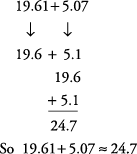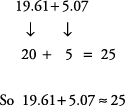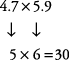## Estimating Sums, Differences, Products, and Quotients

When working with decimals, it is easy to make a simple mistake and misplace the decimal point. Estimating an answer can be a valuable tool in helping you avoid this type of mistake.

#### Estimating sums

Use rounded numbers to estimate sums.

##### Example 1

Give an estimate for the sum of 19.61 and 5.07 by rounding to the nearest tenth.

Round each number to the nearest tenth.##### Example 2

Estimate the sum of 19.61 + 5.07 by rounding to the nearest whole number.

Round each number to a whole number.Estimating differences

Use rounded numbers to estimate differences.

Example 3

Give an estimate for the difference of 12.356 – 5.281 by rounding to the nearest whole number.

Round each number to the nearest whole number.Now subtract.So 12.356 – 5.281 ≈ 7.

#### Estimating products

Use rounded numbers to estimate products.

##### Example 4

Estimate the product of 4.7 × 5.9 by rounding to the nearest whole number.

Round each number to a whole number.So 4.7 × 5.9 ≈ 30.

Again, in decimals, as in whole numbers, if both multipliers end in .5, or are halfway numbers, rounding one number up and one number down will give you a better estimate of the product.

##### Example 5

Estimate the product of 7.5 × 8.5 by rounding to the nearest whole number.You can also round the first number down and the second number up and get this estimate.In either case, your approximation will be closer than it would be if you rounded both numbers up, which is the standard rule.

#### Estimating quotients

Use rounded numbers to estimate quotients.

##### Example 6

Estimate the quotient of 27.49 ÷ 3.12 by rounding to the nearest whole number.

Round each number to the nearest whole number.